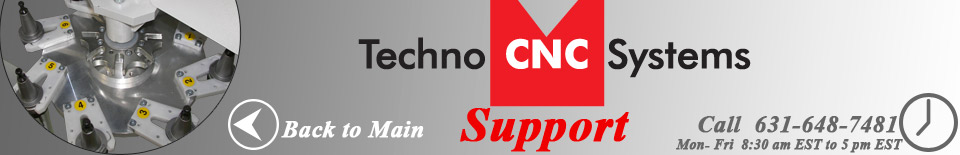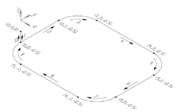The following should help you get familiar with some of the common g-code commands.

## Using Absolute Motion

This program uses absolute motion to cut a four-sided figure with rounded corners.

```G90
M91 OUT1
M91 OUT2
G1 Z-0.50
G1 Y2.00
G2 X1.00 Y3.00 I1.00 J0.00
G1 X4.00
G2 X5.00 Y2.00 I0.00 J-1.00
G1 Y0.00
G2 X4.00 Y-1.00 I-1.00 J0.00
G1 X1.00 Y-1.00
G2 X0.00 Y0.00 I0.00 J1.00
G0 Z0.00
M90 OUT2
M90 OUT1
```Four-sided figure using linear moves and arcs

In this example, the first command indicates that absolute motion will be used to execute the commands that follow. Output 1 and output 2 are turned on. Then, a linear move brings the Z axis down to the -0.5 position. A linear cut is made when the Y axis moves to the +2 position. Next, a clockwise arc is made along the XY plane. When making this arc, the X axis moves to the +1 position, and the Y axis moves to the +3 position. The X and Y axes move clockwise around a center point whose coordinates are 1 unit away in a positive direction along the X axis and the current position of the Y axis.

After the arc is executed, a linear cut is made along the X axis to the +4 position. Then, another clockwise arc is then executed in the XY plane. To make this arc, the X axis moves to the +5 position, and the Y axis moves to the +2 position. The X and Y axes move clockwise around a center point that is equal to the current position of the X axis and is 1 unit away in a negative direction along the Y axis. An additional cutting move brings the Y axis to 0. For the next arc, which is also made in the XY plane, the X axis moves to the +4 position, and the Y axis moves to the -1 position. The X and Y axes move clockwise around a center point that is 1 unit away in a negative direction along the X axis and is equal to the current position of the Y axis. Then, a linear cutting move is executed, bringing the X axis to the +1 position, and the Y axis to the -1 position.

The final arc connects the last linear cut made along the XY axis with the first linear cut. This arc, also a clockwise arc in the XY plane, is created when the X and Y axes both move to the 0 position. The X and Y axes move clockwise around a center point that is equal to the current position of the X axis and is 1 unit away in a positive direction along the Y axis. Then, the Z axis rapids up to the 0 point, and outputs 2 and 1 are turned off.

## Using Incremental Motion

This program uses incremental motion to cut a four-sided figure with rounded corners.

```G91
M91 OUT1
M91 OUT2
G1 Z-0.50
G1 Y2.00
G2 X1.00 Y1.00 I1.00 J0.00
G1 X3.00
G2 X1.00 Y-1.00 I0.00 J-1.00
G1 Y-2.00
G2 X-1.00 Y-1.00 I-1.00 J0.00
G1 X-3.00
G2 X-1.00 Y1.00 I0.00 J1.00
G0 Z0.50
M90 OUT2
M90 OUT1
```Four-sided figure using linear moves and arcs

In the example above, the first command indicates that incremental motion will be used to execute the commands that follow. Output 1 and output 2 are turned on. Then, a linear move brings the Z axis to a position that is 0.5 units away in a negative direction from its current position. A linear cut is made when the Y axis moves 2 units in a positive direction away from its current position. Next, a clockwise arc is made along the XY plane. When making this arc, the X axis moves to a position that is 1 unit away in a positive direction from its current position, and the Y axis moves to a position that is 1 unit away in a positive direction from its current position. The X and Y axes move clockwise around a center point that is 1 unit away in a positive direction along the X axis and is equal to the current position of the Y axis.

After the arc is executed, a linear cut is made along the X axis to a position that is 3 units away in a positive direction from its current position. Then, another clockwise arc is executed in the XY plane. To make this arc, the X axis moves 1 unit away in a positive direction and the Y axis moves 1 unit away in a negative direction from their current positions. The X and Y axes move clockwise around a center point that is equal to the current position of the X axis and is 1 unit away in a negative direction along the Y axis from its current position. A linear cutting move then brings the Y axis to a position that is 2 units away in a negative direction from its current position.

For the next arc, which is also made in the XY plane, the X axis moves 1 unit in a negative direction, and the Y axis moves 1 unit in a negative direction from their current positions. The X and Y axes move clockwise around a center point that is 1 unit away in a negative direction along the X axis and is equal to the current position of the Y axis. Then, a linear cutting move is executed, bringing the X axis to a position that is 3 units away in a negative direction from its current position.

The final arc connects the last linear cut made along the XY axis with the first linear cut. This arc, also a clockwise arc in the XY plane, is created when the X axis moves 1 unit in a negative direction and the Y axis moves 1 unit in a positive direction from their current positions. The X and Y axes move clockwise around a center point that is equal to the current position of the X axis and is 1 unit away in a positive direction along the Y axis. After the arc is completed, the Z axis rapids to a position that is .5 unit away in a positive direction from its current position. Finally, output 2 and then output 1 are turned off.